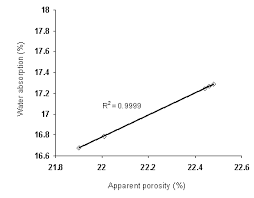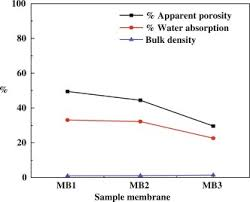## How to Calculate and Solve for Apparent Porosity | Refractories

The apparent porosity is illustrated by the image below.To compute for apparent porosity, three essential parameters are needed and these parameters are Soaked Weight (W), Dried Weight (D) and Suspended Weight (S).

The formula for calulating apparent porosity:

AP = 100((W – D)/(W – S))

Where:

AP = Apparent Porosity
W = Soaked Weight
D = Dried Weight
S = Suspended Weight

Let’s solve an example;
Find the apparent porosity when the soaked weight is 14, the dried weight is 10 and the suspended weight is 12.

This implies that;

W = Soaked Weight = 14
D = Dried Weight = 10
S = Suspended Weight = 12

AP = 100((W – D)/(W – S))
AP = 100((14 – 10)/(14 – 12))
AP = 100(4/2)
AP = 100(2)
AP = 200

Therefore, the apparent porosity is 200%.

## How to Calculate and Solve for Bulk Density | Refractories

The bulk density is illustrated by the image below.To compute for bulk density, four essential parameters are needed and these parameters are Dried Weight (W), Density of Water (ρw), Soaked Weight (W) and Suspended Weight (S).

The formula for calculating bulk density:

BD = w/W – S

Where:

BD = Bulk Density
D = Dried Weight
ρw = Density of Water
W = Soaked Weight
S = Suspended Weight

Let’s solve an example;
Find the bulk density when the dried weight is 6, the density of water is 10, the soaked weight is 14 and the suspended weight is 9.

This implies that;

D = Dried Weight = 6
ρw = Density of Water = 10
W = Soaked Weight = 14
S = Suspended Weight = 9

BD = w/W – S
BD = (6)(10)/(14 – 9)
BD = (60)/(5)
BD = 12

Therefore, the bulk density is 12g/cm³.

Calculating the Dried Weight when the Bulk Density, the Density of Water, the Soaked Weight and the Suspended Weight is Given.

D = BD(W – S) / ρw

Where:

D = Dried Weight
BD = Bulk Density
ρw = Density of Water
W = Soaked Weight
S = Suspended Weight

Let’s solve an example;
Find the dried weight when the bulk density is 10, the density of water is 4, the soaked weight is 8 and the suspended weight is 5.

This implies that;

BD = Bulk Density = 10
ρw = Density of Water = 4
W = Soaked Weight = 8
S = Suspended Weight = 5

D = BD(W – S) / ρw
D = 10(8 – 5) / 4
D = 10(3) / 4
D = 30 / 4
D = 7.5

Therefore, the dried weight is 7.5g.